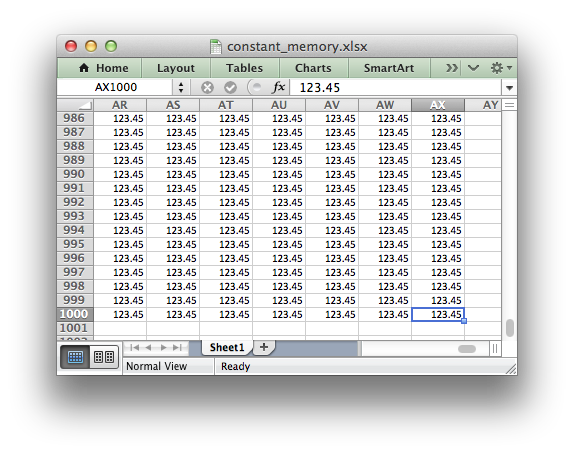libxlsxwriter
constant_memory.c

Example of using libxlsxwriter for writing large files in constant memory mode./*
* Example of using libxlsxwriter for writing large files in constant memory
* mode.
*
* Copyright 2014-2021, John McNamara, jmcnamara@cpan.org
*
*/
#include "xlsxwriter.h"
int main() {
lxw_row_t row;
lxw_col_t col;
lxw_row_t max_row = 1000;
lxw_col_t max_col = 50;
/* Set the worksheet options. */
.tmpdir = NULL,
.use_zip64 = LXW_FALSE};
/* Create a new workbook with options. */
lxw_workbook *workbook = workbook_new_opt("constant_memory.xlsx", &options);
lxw_worksheet *worksheet = workbook_add_worksheet(workbook, NULL);
for (row = 0; row < max_row; row++) {
for (col = 0; col < max_col; col++) {
worksheet_write_number(worksheet, row, col, 123.45, NULL);
}
}
return workbook_close(workbook);
}
workbook_close
lxw_error workbook_close(lxw_workbook *workbook)
Close the Workbook object and write the XLSX file.
lxw_workbook_options::constant_memory
uint8_t constant_memory
Definition: workbook.h:263
lxw_workbook_options
Workbook options.
Definition: workbook.h:261
LXW_FALSE
@ LXW_FALSE
Definition: common.h:51
workbook_new_opt
lxw_workbook * workbook_new_opt(const char *filename, lxw_workbook_options *options)
Create a new workbook object, and set the workbook options.
lxw_worksheet
Struct to represent an Excel worksheet.
Definition: worksheet.h:2107
LXW_TRUE
@ LXW_TRUE
Definition: common.h:53
lxw_workbook
Struct to represent an Excel workbook.
Definition: workbook.h:280
lxw_row_t
uint32_t lxw_row_t
Definition: common.h:40
worksheet_write_number
lxw_error worksheet_write_number(lxw_worksheet *worksheet, lxw_row_t row, lxw_col_t col, double number, lxw_format *format)
Write a number to a worksheet cell.